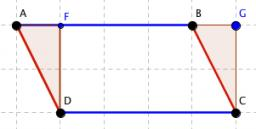# Parallelogram 6288

Find the interior angles of the parallelogram if you know that one of them is 50 degrees larger than the other.

A =  115
B =  65
C =  115
D =  65

### Step-by-step explanation:

A+B = 180
A = B + 50
C = A
D=B

A+B = 180
A-B = 50
A-C = 0
B-D = 0

Row 2 - Row 1 → Row 2
A+B = 180
-2B = -130
A-C = 0
B-D = 0

Row 3 - Row 1 → Row 3
A+B = 180
-2B = -130
-B-C = -180
B-D = 0

Row 3 - -1/-2 · Row 2 → Row 3
A+B = 180
-2B = -130
-C = -115
B-D = 0

Row 4 - 1/-2 · Row 2 → Row 4
A+B = 180
-2B = -130
-C = -115
-D = -65

D = -65/-1 = 65
C = -115/-1 = 115
B = -130/-2 = 65
A = 180-B = 180-65 = 115

A = 115
B = 65
C = 115
D = 65

Our linear equations calculator calculates it.Did you find an error or inaccuracy? Feel free to write us. Thank you!

Tips for related online calculators
Do you have a linear equation or system of equations and looking for its solution? Or do you have a quadratic equation?

#### Grade of the word problem:

We encourage you to watch this tutorial video on this math problem: